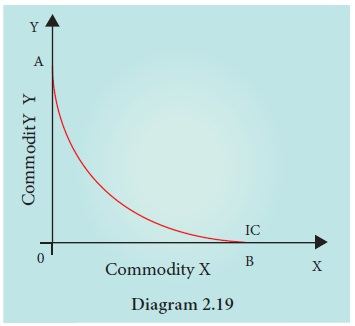Home | | Economics 11th std | Properties of the Indifference Curves

# Properties of the Indifference Curves

Indifference curves are subjective and unique to each person.

Properties of the Indifference Curves

Indifference curves are subjective and unique to each person. Nevertheless they have in common the following properties:

## 1. Indifference curve must have negative slope

An indifference curve has a negative slope, which denotes that if the quantity of commodity (y) decreases, the quantity of the other (x) must increase, if the consumer is to stay on the same level of satisfaction. (a necessary consequence of the non satiety postulate).The curves that do not have negative slopes such as those shown in diagram 2.17 cannot be indifference curves, in all three cases combination B is clearly preferable to combination A.

## 2. Indifference Curves are convex to the origin

Indifference curves are not only negatively sloped, but are also convex to the origin. The convexity of the indifference curves implies that not only the two commodities are substitutes for each other but also the fact that the marginal rate of substitution (MRS) between the goods decreases as a consumer moves along an indifference curve.

## 3. Indifference curve cannot intersectIC1 is lower indifference curve denoting lesser satisfaction. Combination C and B fall on IC1.

IC2 is upper indifference curve denoting higher satisfaction. C and A combinations are on IC2.

At the point of intersection, C=B on IC1 and C=A on IC2. So A=B whereas, A is in upper IC and B is on lower IC. This is not possible.

## 4. Indifference curves do not touch the horizontal or vertical axis.If they touch the axis, it violates the basic assumption that the consumer purchases two commodities in a combination. Purchasing only one commodity means monomania that is consumers’ lack of interest in the other commodity or his insistence on purchasing only one commodity.

Tags : Economics , 11th Economics : Chapter 2 : Consumption Analysis
Study Material, Lecturing Notes, Assignment, Reference, Wiki description explanation, brief detail
11th Economics : Chapter 2 : Consumption Analysis : Properties of the Indifference Curves | Economics